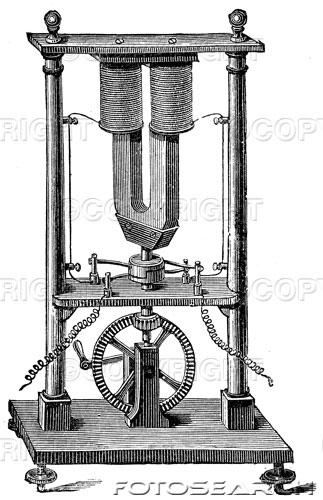` `# Electrical Theory Course Outline

Review of basic Electricity

• Laws of electrical charges
• Attraction and repulsion
• Electrical units of measurement
• Forms of electromotive force

Ohm's Law and D.C. Theory

• Voltage and Power
• Series and Parallel Circuits

A.C. Theory

• Wave forms
• A.C. circuits and Power Factor

Transformers and Generators

• DC Power Sources
• Batteries
• D.C, Generators
• AC Power Sources
• A.C. generators
• Transformers
• Delta and Wye connections

DC and AC Motors

• Types of DC motors and their use
• Types of AC motors and their use
• Wiring characteristics
• Single phase & Poly phase
• Dual voltage motors
• Starting systems
• Basic wiring diagrams